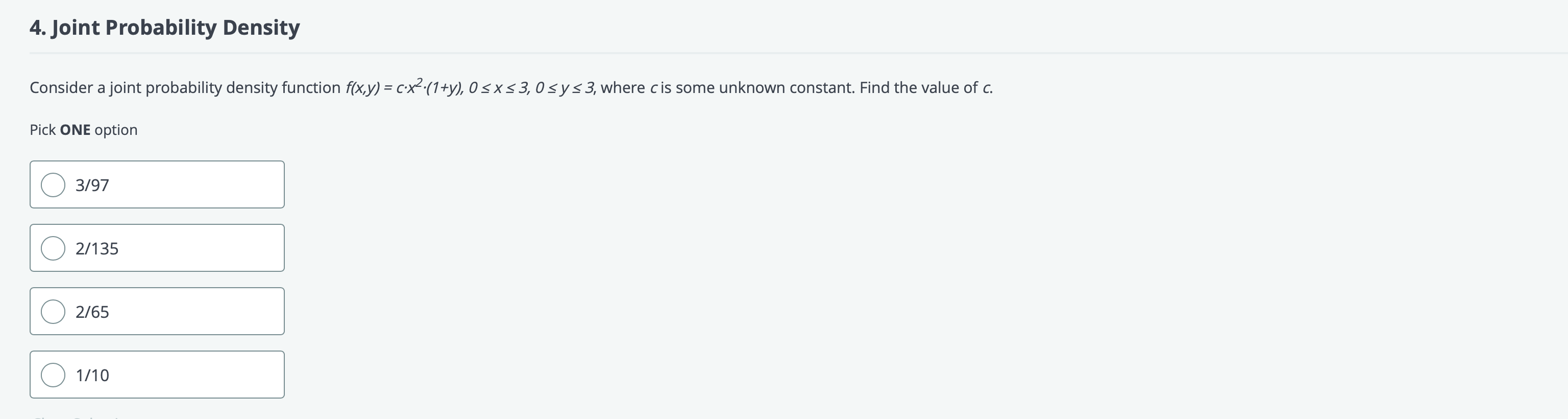# Question Solved1 Answer4. Joint Probability Density Consider a joint probability density function f(x,y) = c.x?:(1+y), O SXS 3,0 s y s3, where cis some unknown constant. Find the value of c. Pick ONE option 3/97 2/135 2/65 1/10PJKG1T The Asker · Probability and StatisticsTranscribed Image Text: 4. Joint Probability Density Consider a joint probability density function f(x,y) = c.x?:(1+y), O SXS 3,0 s y s3, where cis some unknown constant. Find the value of c. Pick ONE option 3/97 2/135 2/65 1/10
More
Transcribed Image Text: 4. Joint Probability Density Consider a joint probability density function f(x,y) = c.x?:(1+y), O SXS 3,0 s y s3, where cis some unknown constant. Find the value of c. Pick ONE option 3/97 2/135 2/65 1/10